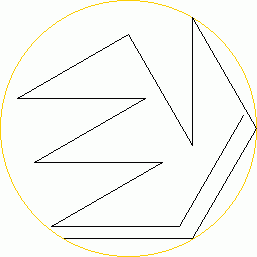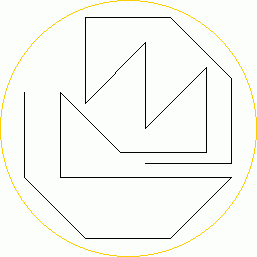# Al Zimmermann's Programming Contests

## Snakes On A Plane

### by Al Zimmermann

#### based upon an idea by Serhiy Grabarchuk

Introduction

Find the longest possible path that fits into a circle of given size.
This problem is originally known as "Matchstick snake problem".
See http://www.stetson.edu/%7Eefriedma/mathmagic/0503.html

For every pair (N,D), you should submit the longest possible path, described by the sequence of turning angles between consecutive segments.
D is an integer such that the whole path will fit inside a circle of diameter D. The path is allowed to touch the circle.
N is the integer number characterizing the set of N-1 possible turning angles between consecutive segments.
Here are the different values of N and D for this contest:
 N D 5 3 4 5 6 7 8 9 10 11 12 13 14 7 2 3 4 5 6 7 8 9 10 11 8 2 3 4 5 6 7 8 9 10 9 2 3 4 5 6 7 8 9 10 2 3 4 5 6 7 8 11 2 3 4 5 6 7 12 3 4 5 6 7
The path is composed of a sequence of straight line segments, each 1 unit long.
The path may neither intersect nor touch itself anywhere, except for the last segment, which can finish at the starting point. In this case, the path is closed.
You have to enter the value of N, but you don't have to mention the value of D.

You can submit your paths in two formats:
1. N followed by a sequence of integer numbers.
The sequence of submitted numbers is the sequence of k values, where k is an integer from 1 to N-1.
At the end of every segment the path turns 180(2k/N - 1) degrees.
(Note that if k = N/2 the path turns 0 degrees; that is, it continues straight ahead.)

An example:
```12 8 8 11 1 9 11 1 11 1 11 8
```
gives:You submitted: 12 8 8 11 1 9 11 1 11 1 11 8 N=12 Found 12 valid segments Radius=1.000000000000000000000000000000 X start=-0.500000000000000000000000000000 Y start=-0.866025403784438646763723170753 Diameter = 2.000000000000000000000000000000 Rounded to 2 Score(12,2) = 12
Note: you can submit this solution as 12 BBEeCEeEeEB with the other format.

2. N followed by a sequence of characters.
0 ... means straight ahead (only possible for even N)
A,B,C,... mean a counter-clockwise turn.
a,b,c,... mean a clockwise turn.

Below are the values of all the possible angles in degrees:
N=5: -36(a), +36(A), -108(b), +108(B)
N=7: approx. -25.7(a), +25.7(A), -77.1(b), +77.1(B), -128.6(c),+128.6(C)
N=8: 0(0), -45(a), +45(A), -90(b), +90(B), -135(c), +135(C)
N=9: +-20(Aa), +-60(Bb), +-100(Cc), +-140(Dd)
N=10: 0(0), +-36(Aa), +-72(Bb), +-108(Cc), +-144(Dd)
N=11: approx. +-16.36(Aa), +-49.1(Bb), +-81.82(Cc), +-114.55(Dd), +-147.27(Ee)
N=12: 0(0), +-30(Aa), +-60(Bb), +-90(Cc), +-120(Dd), +-150(Ee)

In the path computation the approximate angles given in the table for N=7 and N=11 are to be replaced by the corresponding exact values that are the nearest matching multiples of (180/N).

An example:
```8 BAABCcCcbaCB0caaa

```
3. gives:You submitted: 8 BAABCcCcbaCB0caaa N=8 Found 18 valid segments Radius=1.386208092334556349669864212738 X start=0.207106781186547524400844362105 Y start=-0.414213562373095048801688724210 Diameter = 2.772416184669112699339728425476 Rounded to 3 Score(8,3) = 18
Note: you can submit this solution as 8 6 5 5 6 7 1 7 1 2 3 7 6 4 1 3 3 3 with the other format.

The Scoring System

When you submit a solution for a given N, the scorer will compute the diameter D of the smallest enclosing circle and round it to the next bigger or equal integer diameter.
Your score for this pair (N,D) will be equal to:
Score(N,D)=(your submitted number of segments)/(best submitted number of segments)

Total Scoring

Your total score is the sum of all single problem scores for pairs (N,D).
Since there are 57 pairs, the best total score you can get is 57.
If you do not submit for a particular pair (N,D), you will receive a 0 for that pair.

Prizes

First prize is \$240, second prize is \$100.
In case of a tie, the entrant who reached the tied score first wins.
At the end of every week for the first 10 weeks, the score leader will win \$10.
There will be four special prizes of \$15 for the longest closed paths for N=9, N=10, N=11 and N=12.
All prizes will be paid via Paypal.

Organization

As the organizers, we may change the rules during this contest.

Thanks

Special thanks to Hugo Pfoertner, James Buddenhagen, Dmitry Kamenetsky, Klaus Nagel, Jaroslaw Wroblewski, Al Zimmermann, Juha Saukkola, Fred Mellender and Mark Beyleveld for helping setting up the rules and beta-testing the scorer.